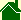#Overview of what time is

Hello, my name is John Thomas Nordberg. I claim I can answer the question, "What is time?" To summarize what time is:

• Time is a specific motion.

• People use this specific motion as a standard of motion to measure all other motions. (Just as people use a standard of length to measure other lengths.)

• Through history, the specific motion people have used to measure other motions -- the motion people to this day still call "time" -- is the apparent motion of the sun as it crosses the sky for an earthbound observer.

• I claim that using the sun's motion as a standard for measuring other motions is a major scientific mistake. The reason is, if you move a clock -- which is just a representation of the sun's motion -- the clock develops an error. This error is a result of the motion of the clock combining with the motion on the clock.

• I claim that Einstein's Theory of Relativity is only a correction factor that compensates for the errors caused by moving a classic timepiece. In other words, the mistake every physicist is making is: they use the sun's motion as their standard of motion and they use the correction factor called "Relativity" when they use a moving a clock as their technique to measure all other motions. Motions must not be measured by using time in this way. (See also, Proof Relativity is Illogical)

• Instead, time must be defined as the fastest known motion -- presently, what we now call the "speed of light." All other motions should be represented as fractions of this motion.
• To correctly measure a velocity or a speed, scientists must divide the distance an object travels by the distance light travels.

• To summarize: time is a constant quantity of motion, that is used to measure other motions, and should be defined to be what we now call the speed of light in free space. Time is the speed of light. The speed of light is time. To correctly measure a velocity or a speed, scientists must divide the distance an object travels by the distance light travels. A simple summary of the scientific problem of time is: scientists currently define the "speed of light" in terms of the sun's apparent motion. Instead, the sun's apparent motion must be defined in terms of "the speed of light."